# Persistent State of Packages in Oracle

## Persistent State of Packages in Oracle

In this article, I am going to discuss the Persistent State of Packages in Oracle with Examples. Please read our previous article where we discussed Forward Declaration in Oracle. If we try to learn about the persistent state of packages is very difficult. The best way to understand is directly run with the exercise and see how it is defined.

##### Example to Understand Persistent State of Packages in Oracle

We will take a simple package with a persistent_state name.

```create or replace package Persistent_state
is
g_var number:=10;
procedure update_g_var ( p_no number);
end;
```

We can see the package name is persistent_state where the defined variable g_var with value 10. This value is a global variable and the default value is 10. We will declare a procedure update_g_var which takes one parameter as the input. Let’s now try to compile the package specification.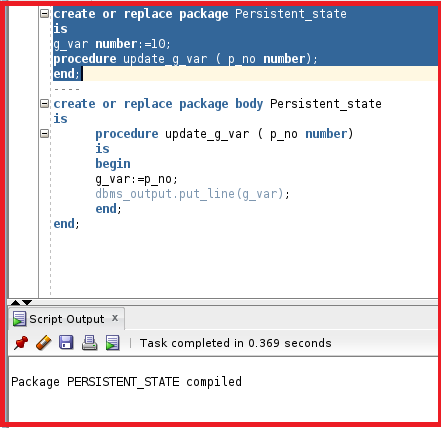The package specification is now compiled. Let’s have a look at the package body.

```create or replace package body Persistent_state
is
procedure update_g_var ( p_no number)
is
begin
g_var:=p_no;
dbms_output.put_line(g_var);
end;
end;
```

The package body contains the procedure update_g_var which takes p_no as the input number. We will assign the p_no to the global variable g_var. We will print the variable g_var using dbms_output. Let’s compile the package body.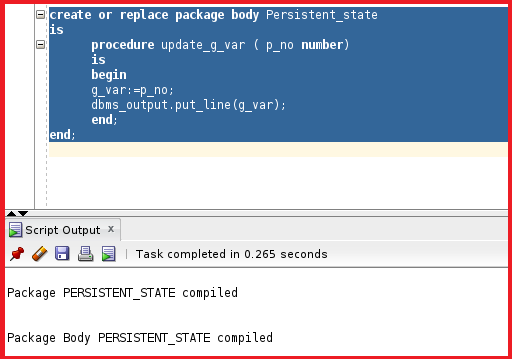So, the package body PERSISTENT_STATE is now compiled. Let’s try to test the package by running the procedure inside the package.

execute Persistent_state.update_g_var(80);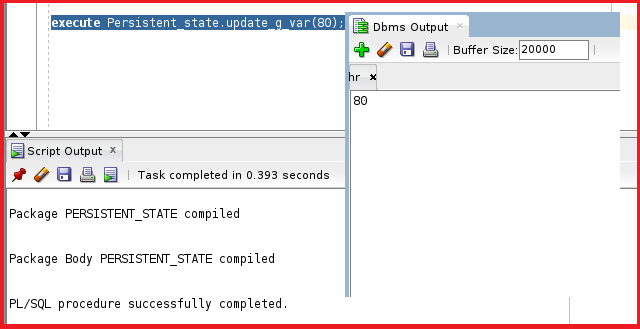So, the procedure is now executed successfully. We got the DBMS output as 80. This is the same parameter that we have given in the input.

So, now we need to understand the persistent state. Previously while creating the package specification the value of g_var is defined as 80. But when we assigned the g_Var variable with p_no then the value of g_var is changed to p_no. In order to prove that let’s take another variable as a test and assign the g_Var value to the test variable.

```Variable test number;
execute :test:=Persistent_state.g_var;```

Now, this execute statement will assign the g_Var value to the test variable. Let’s try to execute this statement.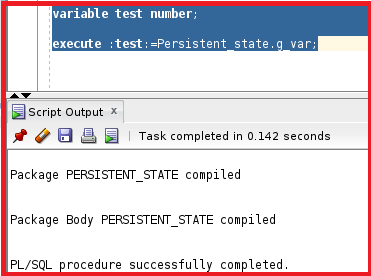So, the statement is executed successfully. Let’s try to check the value of the test variable.

print test;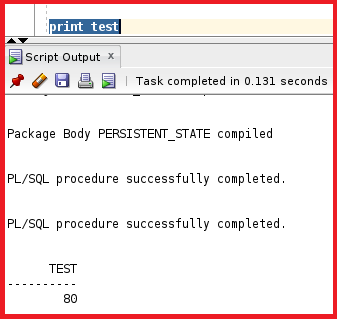So, the variable test has the value 80 which is assigned from g_var. Let’s try to do another test to see the value of g_var.

```declare
x number;
begin
x:=Persistent_state.g_var;
dbms_output.put_line (x);
end;
```

Here we have declared a variable x with datatype as a number. We have assigned the g_var value to the x variable. The value of x will be printed with dbms_output. Let’s try to execute this statement.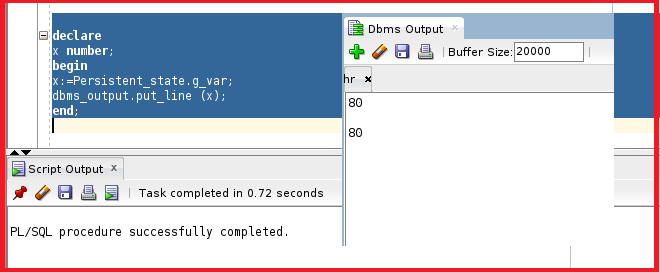After executing the above statement, the variable x is also assigned from the variable 80. So, the value of variable g_var had changed from 10 to 80.

Note: The value 80 is only unique for this session and the value will be terminated once the session is terminated. So, the package body contains the procedure which takes a parameter as the input and assigns the value to the global variable g_var, and displays the g_var value in the dbms_output.

##### Persistent State in Oracle:

The collection of package variables and the values define the package state. The package is

• Initialized when the package is first loaded.
• Persistent for the life of the session.
1. Stored in the User Global Area(UGA). So, the value 80 is stored in UGA.
2. This UGA is unique to each session
3. Subject to change when package subprograms are called or public variables are modified.
• Not persistent for the session but persistent for the life of a subprogram call when using PRAGMA SERIALLY_REUSABLE in the package specification.

In the next article, I am going to discuss PRAGMA SERIALLY_REUSABLE in Oracle with Examples. Here, in this article, I try to explain the Persistent State of Packages in Oracle with Examples. I hope you enjoy this Persistent State of Packages in Oracle article.